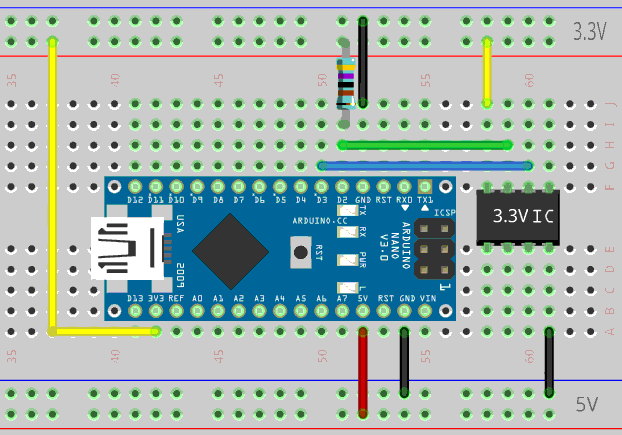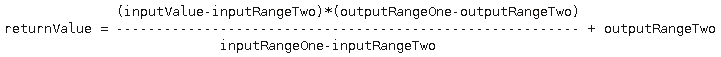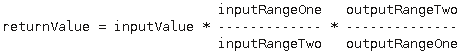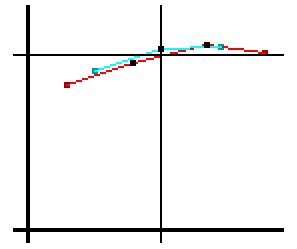I think the one consensus about Arduino is that the build environment and the library suck.  That said, I use it.  This is some code I have written to try to make it a bit better for at least the limited things that I do with it.

For this post, I’m assuming you know how to use the Arduino IDE and how to program in it.  I make no effort to teach programming here and just present the code.

Diving right in with one of the worst: digitalWrite()

http://pastebin.com/dSRSxgax is a friend’s example of just how bad it is compared to harder to read but faster and smaller code.

My solution:  A macro that compiles down to an sbi or cbi.  All ATmega328 series boards should work, but I only tested on the Nano.  Comments with patches for other boards will be considered.

A quick look at the schematic shows the following ports:

```  D0-D7:PORTD
D8-D13:PORTB
A0-A7:PORTC
```

For example:

```  #define OutputPin A5
digitalWrite(OutputPin, HIGH);```

is far more efficient as:

```  #define OutputPin A5
PORTC |= (1<<OutputPin-A0);```

The following are macros for both read and write that do the above but work for any pin and also include a pinMode() replacement: (not my code)

```#define digitalPinToPortReg(P) (((P) >= 0 && (P) <= 7) ? &PORTD : (((P) >= 8 && (P) <= 13) ? &PORTB : &PORTC))
#define digitalPinToPINReg(P) (((P) >= 0 && (P) <= 7) ? &PIND : (((P) >= 8 && (P) <= 13) ? &PINB : &PINC))
#define digitalPinToBit(P) (((P) >= 0 && (P) <= 7) ? (P) : (((P) >= 8 && (P) <= 13) ? (P) - 8 : (P) - 14))
#define digitalPinToDDRReg(P) (((P) >= 0 && (P) <= 7) ? &DDRD : (((P) >= 8 && (P) <= 13) ? &DDRB : &DDRC))
#define pinModeFast(P, V) bitWrite(*digitalPinToDDRReg(P), digitalPinToBit(P), (V))
#define digitalWriteFast(P, V) bitWrite(*digitalPinToPortReg(P), digitalPinToBit(P), (V))

This simple to use set of macros assumes PWM and timers are not in use on the related pins and it will fail to compile if the pin (P) isn’t a constant. Variables of type const will not work, #define does work.
These are all things the programmer can keep in mind while writing their code and are hardly a burden.  If not all of the above apply, use the bigger, slower digitalWrite() instead.

Simplified Level Shifting is also possible.  The usual voltage divider or transistor to write to a 3.3V device requires at least two components and in the case of the voltage divider, will create a poor quality signal.  The method described here only requires a single resistor.
There is a caveat, you must burn the code before connecting the hardware and if you make a mistake in your code, you can damage your hardware.
The trick here is that to output a HIGH to a 3.3V device we actually set the port to INPUT and use an external 4.7K pull-up resistor to 3.3V.  To output a low, the pin is set to LOW and the pinMode is set to OUTPUT.

DO NOT use pinModeFast(pin, OUTPUT) or pinMode(pin, OUTPUT) with this function.

Assuming D2 is and output and D3 is an input, the hardware would be as shown:
The code to handle simplified level shifting is:`#define digitalWriteLevelShift(P, V) (V) ? bitClear(*digitalPinToDDRReg(P), digitalPinToBit(P)) : (bitClear(*digitalPinToPortReg(P),digitalPinToBit(P));bitSet(*digitalPinToDDRReg(P), digitalPinToBit(P)))`

This macro depends on the macros from the section above.  To use it, do nothing in setup(), do not use the usual pinMode(pin, OUTPUT); call.  Simply use digitalWriteLevelShift(pin,HIGH); or digitalWriteLevelShift(pin,LOW);.  As with the other functions, V can be a variable but pin (P) must meet all the restrictions for the other macros above.  For P, variables of type const will not work, #define does work.

An even bigger speedup can come from improving the way the ADC is read.  With default settings, the Arduino library sits doing nothing for about 110uS while waiting for the ADC to convert, that’s a very large chunk of time that could be used to do other things.

This one isn’t just limited to the Arduino library, the majority of code I’ve seen for all chips is done the same way.  There is another option:

Split the ADC read into 3 parts, Start, Poll, and Read.  To use it, you start a conversion, then continue doing whatever else you want, and poll whenever convenient to see if it’s done.  When the polling says it’s done, you read the result.  Usually you’ll start the first conversion in setup() and after each result, you’ll start the next reading.  The overall improvement is a bit hard to quantify but the startSample() call takes 2.1uS, the sampleDone() check takes 1.7uS and the getSampleResult() call takes 1.8uS leaving your code more than 100uS more time per ADC read to do calculations or to speed it’s main loop.  This might allow you to get your response latency for other things down to an acceptable level without resorting to interrupts and thereby simplify your system.  If you actually need the ADC to do more conversions per second, see part 2 of this article (referenced below the code.)

```#include "wiring_private.h"
#include "pins_arduino.h"

void startSample(uint8_t pin){
uint8_t analog_reference = DEFAULT;
#if defined(analogPinToChannel)
#if defined(__AVR_ATmega32U4__)
if (pin >= 18) pin -= 18; // allow for channel or pin numbers
#endif
pin = analogPinToChannel(pin);
#elif defined(__AVR_ATmega1280__) || defined(__AVR_ATmega2560__)
if (pin >= 54) pin -= 54; // allow for channel or pin numbers
#elif defined(__AVR_ATmega32U4__)
if (pin >= 18) pin -= 18; // allow for channel or pin numbers
#elif defined(__AVR_ATmega1284__) || defined(__AVR_ATmega1284P__) || defined(__AVR_ATmega644__) || defined(__AVR_ATmega644A__) || defined(__AVR_ATmega644P__) || defined(__AVR_ATmega644PA__)
if (pin >= 24) pin -= 24; // allow for channel or pin numbers
#else
if (pin >= 14) pin -= 14; // allow for channel or pin numbers
#endif
// the MUX5 bit of ADCSRB selects whether we're reading from channels
// 0 to 7 (MUX5 low) or 8 to 15 (MUX5 high).
ADCSRB = (ADCSRB & ~(1 << MUX5)) | (((pin >> 3) & 0x01) << MUX5);
#endif
// set the analog reference (high two bits of ADMUX) and select the
// to 0 (the default).
ADMUX = (analog_reference << 6) | (pin & 0x07);
#endif
// without a delay, we seem to read from the wrong channel
//delay(1);
// start the conversion
#endif
}
```
```uint8_t sampleDone(){
// ADSC is cleared when the conversion finishes
}
```
```uint16_t getSampleResult(){
uint8_t low, high;
// cause the results of each conversion to be discarded,
#else
// we dont have an ADC, return 0
low = 0;
high = 0;
#endif
// combine the two bytes
return (high << 8) | low;
}
```

Further improvements to ADC performance are in my post: Speeding up the ADC on an Arduino ATMega 328P

Next up is code for doing linear conversions and scaling.

This is similar to Arduino’s:

`long map(long x, long in_min, long in_max, long out_min, long out_max)`

The version presented here  doesn’t simply throw longer integers at the problem and it avoids doing everything with slower 32 bit math.

scale(): Effectively this is the same as a units conversion equation with support for different zero points such as the celsius conversion described at http://oakroadsystems.com/math/convert.htm
The equation is arranged as below to reduce lost precision from using integers by doing the division as late as possible.  The simple form should be familiar from 9th grade chemistry class.The simple form of this equation is:or solved for returnValue:EXAMPLE 1: Fahrenheit to Celsius in integer

```#define Extrapolate
int16_t c = scale(f,32,212,0,100);```

//c=((70-212)*(0-100))/(32-212)+100 //70F randomly chosen
//c=(-142*-100)/-180+100
//c=14200/-180+100 //notice 14200 is quite large, that is the reason for the 32bit cast.
//c=-78+100
//c=22 //notice the error due to integer math, 21.111… is more accurate but to get that would require slower math.

EXAMPLE 2: Fahrenheit to Celsius in fixed point math (100ths of F -> 100ths of C)

```#define Extrapolate
int16_t c = scale(f,3200,21200,0,10000);```

//c=((7000-21200)*(0-10000))/(3200-21200)+10000 //70.00F randomly chosen
//c=(-14200*-10000)/-18000+10000
//c=142000000/-18000+10000 //notice 142,000,000 is quite large, that is the reason for the 32bit cast.
//c=-7888+10000
//c=2112 //21.12C is still a bit off but is much closer and runs without using much slower floating point math.

EXAMPLE 3:  Time correcting with linear iterpolationWhen correlating two sample sets, you often find that the offset time indexes don’t line up correctly.  If the data is compatible, often you can graph the samples on Y with time on X and draw a line between the closest two points, then find the intersection with the offset time of the sample in the other data set.  The image to the right shows an example group of data points from two sample sets graphed against time.  The 3 black points are the ones we’re using.

The process could also be used to take virtual samples at specific offset times, creating approximate data with regular intervals out of measured data at irregular intervals.

`redVirtualSample=scale(blueSampleTime,redSampleTime,redSampleTime,redSample,redSample);`

If the samples are:

Red 19,72  Blue 33,79
Red 52,83  Blue 66,90
Red 89,93  Blue 96,91
Red 118,89

Then the above becomes:

```redVirtualSample=scale(66,52,89,83,93);
redVirtualSample=((66-89)*(83-93))/(52-89)+93
redVirtualSample=(-23*-10)/-37+93
redVirtualSample=230/-37+93
redVirtualSample=86```

Looking at the graph, we can visually observe that this is about right.

Without further ado, here is the code:

```int16_t scale( int16_t inputValue,
int16_t inputRangeOne,
int16_t inputRangeTwo,
int16_t outputRangeOne,
int16_t outputRangeTwo){
//#define Extrapolate //define if values outside of inputRangeOne to inputRangeTwo are permitted
#ifndef Extrapolate
//special case for input at end of range or out of range.
// equation supports inverting (higher inputs result in lower outputs) so
// detect that first to allow propper out/end of range detection
if(inputRangeOne>inputRangeTwo){ //not input inverted
if(inputValue>=inputRangeOne) return outputRangeOne;
if(inputValue<=inputRangeTwo) return outputRangeTwo;
}else{ //input inverted
if(inputValue<=inputRangeOne) return outputRangeOne;
if(inputValue>=inputRangeTwo) return outputRangeTwo;
}
#endif
//main equation as described above
return ((int32_t)((int16_t)inputValue-inputRangeTwo)*((int16_t)outputRangeOne-outputRangeTwo))/(inputRangeOne-inputRangeTwo)+outputRangeTwo;
}```

More functions and macros will be added to this post over time.

“the breadboard image above was created with Fritzing”